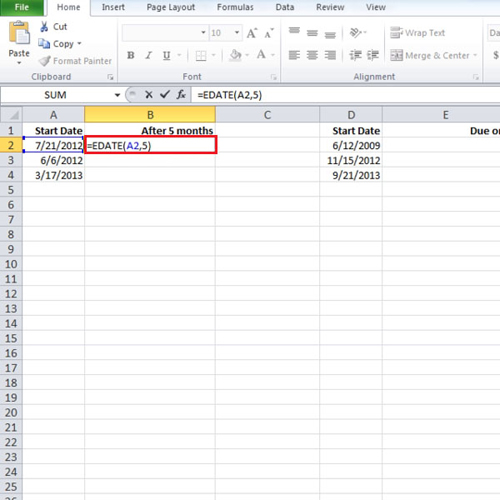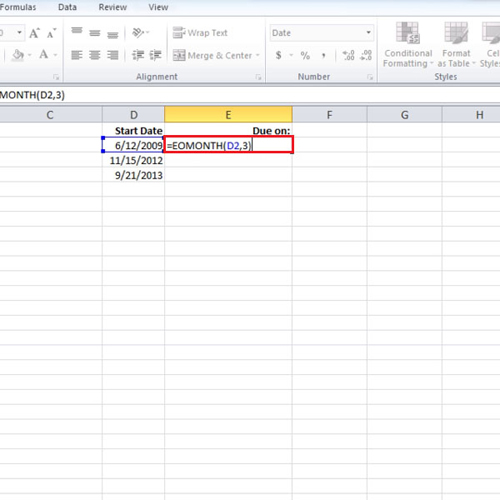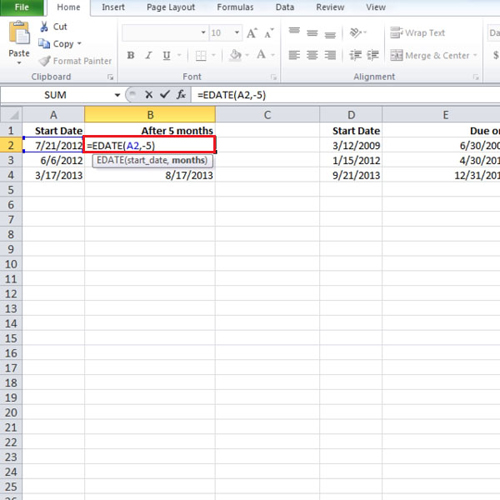## How to calculate ends of months and future or past dates in Excel

There are several functions in Excel with help you in various ways. These functions are not all related to calculations. Calculating end of month dates in Excel is done by using the edate fuction. This edate function calculates end of month dates in Excel from the specific month you input. For example if one needs to find out what will be the date five months from now, this function is used.
In this tutorial, we will teach you how to calculate end of months and future or past dates in Excel.

Step # 1 – Insert Edate function
Suppose, if you want to find the date after a certain number of years, then you can use the “edate” function. Usually when one needs to find out what will be the date five months from now, this function is used. Type:
= edate(a2,5)
In this tutorial, we typed cell “a2” because the starting date is in cell “a2” and since we want to know the date 5 months from now, we entered “5” in the function. You can use the auto fill option to fill the rest of the dates in the column.Step # 2 – Apply Eomonth function
There is another function in Excel which can be used to find out the end of the month date.
In this tutorial, in column “d” we have some more dates and we want to find out what will be the last date of the month, three months from now. Type:
=eomonth(d2,3)Step # 3 – Modify the function
If you change the start date, the calculated date will be adjusted accordingly.
Let us say, you want to know the date which was five months ago from now. Simply use a “negative” sign in the formula before the number. So we used a negative sign before “five” and hence the calculation was done accordingly. These functions in Excel save you all the time that you would have otherwise spent calculating the dates manually with the calendar in front of you.